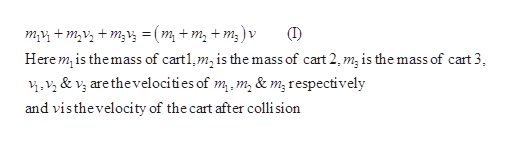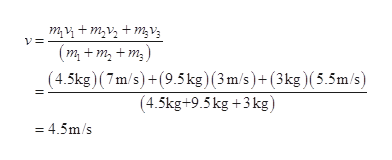# Three carts of masses m1 = 4.50 kg, m2 = 9.50 kg, and m3 = 3.00 kg move on a frictionless, horizontal track with speeds of v1 = 7.00 m/s to the right, v2 = 3.00 m/s to the right, and v3 = 5.50 m/s to the left, as shown below. Velcro couplers make the carts stick together after colliding.(a) Find the final velocity of the train of three carts.magnitude m/sdirection (b) Does your answer require that all the carts collide and stick together at the same moment?     What if they collide in a different order?

Question
84 views

Three carts of masses m1 = 4.50 kg, m2 = 9.50 kg, and m3 = 3.00 kg move on a frictionless, horizontal track with speeds of v1 = 7.00 m/s to the right, v2 = 3.00 m/s to the right, and v3 = 5.50 m/s to the left, as shown below. Velcro couplers make the carts stick together after colliding.

(a) Find the final velocity of the train of three carts.

 magnitude m/s direction

(b) Does your answer require that all the carts collide and stick together at the same moment?

What if they collide in a different order?

check_circle

Step 1

a)

According to law of conservation of linear momentum,help_outlineImage Transcriptionclose(I) m, + m,v, + m;V; = (m, + m, + m; )v Here m, is themass of cart1,m, is the mass of cart 2, m, is the mass of cart 3, i.v, & v, arethevelocities of m. m, & m; respectively and visthevelocity of the cart after colli sion fullscreen
Step 2

Substitute the values in (I) and rearrange in terms of vhelp_outlineImage Transcriptionclosem,V + m,V, + m;V; (m + m, + m;) v= (4.5kg) (7m/s)+(9.5 kg) (3m/s)+(3kg)(5.5m/s) (4.5kg+9.5kg +3 kg) = 4.5m/s fullscreen
Step 3

The final velocity is 4.5...

### Want to see the full answer?

See Solution

#### Want to see this answer and more?

Solutions are written by subject experts who are available 24/7. Questions are typically answered within 1 hour.*

See Solution
*Response times may vary by subject and question.
Tagged in

### Newtons Laws of Motion